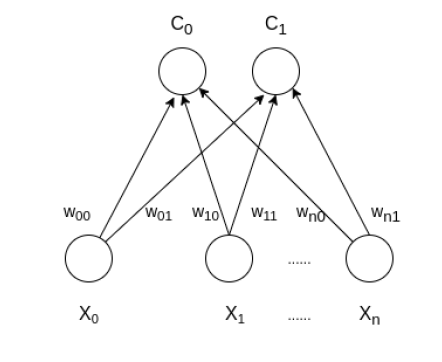Open in App
Not now

# Self Organizing Maps – Kohonen Maps

• Difficulty Level : Hard
• Last Updated : 02 Jan, 2023

Self Organizing Map (or Kohonen Map or SOM) is a type of Artificial Neural Network which is also inspired by biological models of neural systems from the 1970s. It follows an unsupervised learning approach and trained its network through a competitive learning algorithm. SOM is used for clustering and mapping (or dimensionality reduction) techniques to map multidimensional data onto lower-dimensional which allows people to reduce complex problems for easy interpretation. SOM has two layers, one is the Input layer and the other one is the Output layer. The architecture of the Self Organizing Map with two clusters and n input features of any sample is given below:## How do SOM works?

Let’s say an input data of size (m, n) where m is the number of training examples and n is the number of features in each example. First, it initializes the weights of size (n, C) where C is the number of clusters. Then iterating over the input data, for each training example, it updates the winning vector (weight vector with the shortest distance (e.g Euclidean distance) from training example). Weight updation rule is given by :

`wij = wij(old) + alpha(t) *  (xik - wij(old))`

where alpha is a learning rate at time t, j denotes the winning vector, i denotes the ith feature of training example and k denotes the kth training example from the input data. After training the SOM network, trained weights are used for clustering new examples. A new example falls in the cluster of winning vectors.

### Algorithm

Training:

Step 1: Initialize the weights wij random value may be assumed. Initialize the learning rate α.

Step 2: Calculate squared Euclidean distance.

D(j) = Σ (wij – xi)^2    where i=1 to n and j=1 to m

Step 3: Find index J, when D(j) is minimum that will be considered as winning index.

Step 4: For each j within a specific neighborhood of j and for all i, calculate the new weight.

wij(new)=wij(old) + α[xi – wij(old)]

Step 5: Update the learning rule by using :

α(t+1) = 0.5 * t

Step 6: Test the Stopping Condition.

Below is the implementation of the above approach:

## Python3

 `import` `math`   `class` `SOM:`   `    ``# Function here computes the winning vector` `    ``# by Euclidean distance` `    ``def` `winner(``self``, weights, sample):`   `        ``D0 ``=` `0` `        ``D1 ``=` `0`   `        ``for` `i ``in` `range``(``len``(sample)):`   `            ``D0 ``=` `D0 ``+` `math.``pow``((sample[i] ``-` `weights[``0``][i]), ``2``)` `            ``D1 ``=` `D1 ``+` `math.``pow``((sample[i] ``-` `weights[``1``][i]), ``2``)`   `            ``if` `D0 > D1:` `                ``return` `0` `            ``else``:` `                ``return` `1`   `    ``# Function here updates the winning vector` `    ``def` `update(``self``, weights, sample, J, alpha):`   `        ``for` `i ``in` `range``(``len``(weights)):` `            ``weights[J][i] ``=` `weights[J][i] ``+` `alpha ``*` `(sample[i] ``-` `weights[J][i])`   `        ``return` `weights`   `# Driver code`     `def` `main():`   `    ``# Training Examples ( m, n )` `    ``T ``=` `[[``1``, ``1``, ``0``, ``0``], [``0``, ``0``, ``0``, ``1``], [``1``, ``0``, ``0``, ``0``], [``0``, ``0``, ``1``, ``1``]]`   `    ``m, n ``=` `len``(T), ``len``(T[``0``])`   `    ``# weight initialization ( n, C )` `    ``weights ``=` `[[``0.2``, ``0.6``, ``0.5``, ``0.9``], [``0.8``, ``0.4``, ``0.7``, ``0.3``]]`   `    ``# training` `    ``ob ``=` `SOM()`   `    ``epochs ``=` `3` `    ``alpha ``=` `0.5`   `    ``for` `i ``in` `range``(epochs):` `        ``for` `j ``in` `range``(m):`   `            ``# training sample` `            ``sample ``=` `T[j]`   `            ``# Compute winner vector` `            ``J ``=` `ob.winner(weights, sample)`   `            ``# Update winning vector` `            ``weights ``=` `ob.update(weights, sample, J, alpha)`   `    ``# classify test sample` `    ``s ``=` `[``0``, ``0``, ``0``, ``1``]` `    ``J ``=` `ob.winner(weights, s)`   `    ``print``(``"Test Sample s belongs to Cluster : "``, J)` `    ``print``(``"Trained weights : "``, weights)`     `if` `__name__ ``=``=` `"__main__"``:` `    ``main()`

Output:
Test Sample s belongs to Cluster : 0
Trained weights : [[0.6000000000000001, 0.8, 0.5, 0.9], [0.3333984375, 0.0666015625, 0.7, 0.3]]

My Personal Notes arrow_drop_up
Related Articles